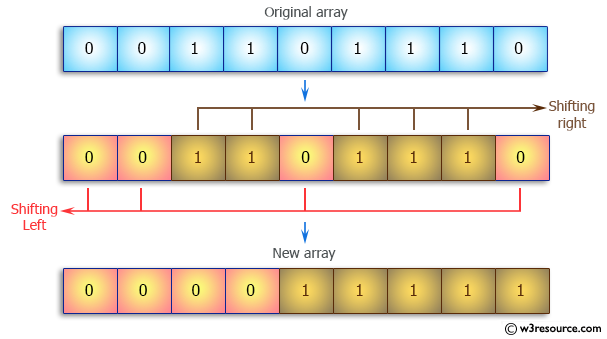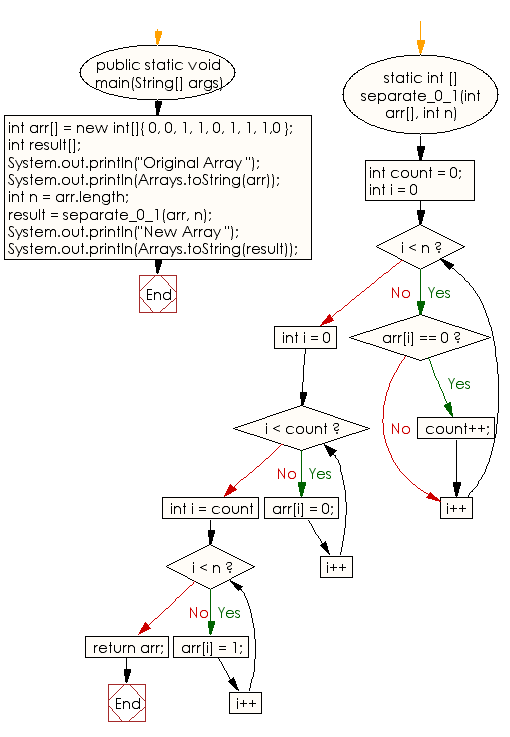﻿ Java: An array with 0s on the left and 1s on the right# Java Array Exercises: Separate 0s on left side and 1s on right side of an array of 0s and 1s in random order

## Java Array: Exercise-51 with Solution

Write a Java program to separate 0s on left side and 1s on right side of an array of 0s and 1s in random order.

Pictorial Presentation:Sample Solution:

Java Code:

``````import java.util.Arrays;

public class Main {
public static void main(String[] args)
{
int arr[] = new int[]{ 0, 0, 1, 1, 0, 1, 1, 1,0 };
int result[];
System.out.println("Original Array ");
System.out.println(Arrays.toString(arr));

int n = arr.length;

result = separate_0_1(arr, n);
System.out.println("New Array ");
System.out.println(Arrays.toString(result));
}

static int [] separate_0_1(int arr[], int n)
{
int count = 0;

for (int i = 0; i < n; i++) {
if (arr[i] == 0)
count++;
}

for (int i = 0; i < count; i++)
arr[i] = 0;

for (int i = count; i < n; i++)
arr[i] = 1;

return arr;
}
}
```
```

Sample Output:

```
Original Array
[0, 0, 1, 1, 0, 1, 1, 1, 0]
New Array
[0, 0, 0, 0, 1, 1, 1, 1, 1]
```

Flowchart:Visualize Java code execution (Python Tutor):

Java Code Editor:

Improve this sample solution and post your code through Disqus

What is the difficulty level of this exercise?

Test your Programming skills with w3resource's quiz.

﻿

## Java: Tips of the Day

countOccurrences

Counts the occurrences of a value in an array.

Use Arrays.stream().filter().count() to count total number of values that equals the specified value.

```public static long countOccurrences(int[] numbers, int value) {
return Arrays.stream(numbers)
.filter(number -> number == value)
.count();
}
```

Ref: https://bit.ly/3kCAgLb